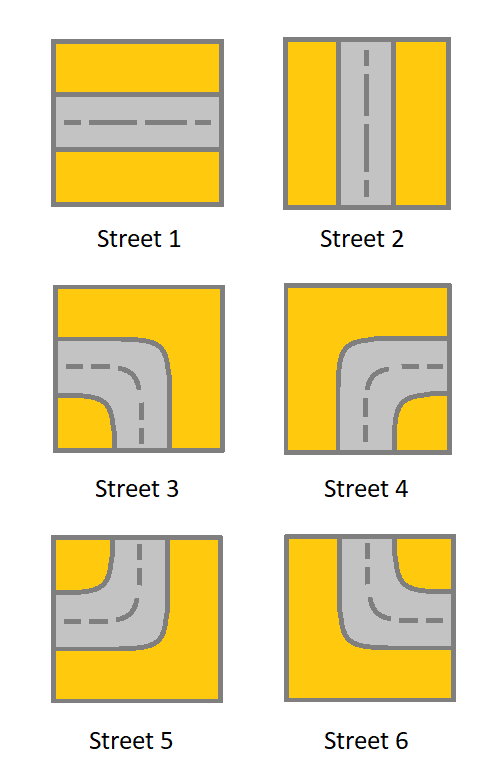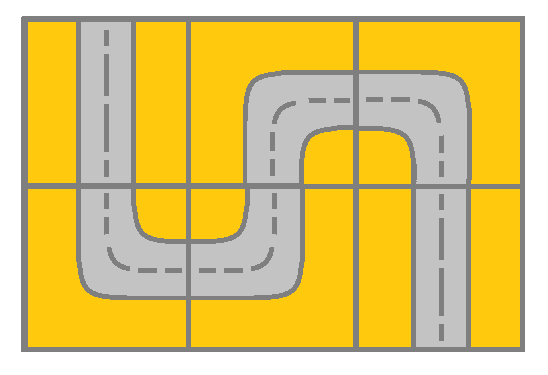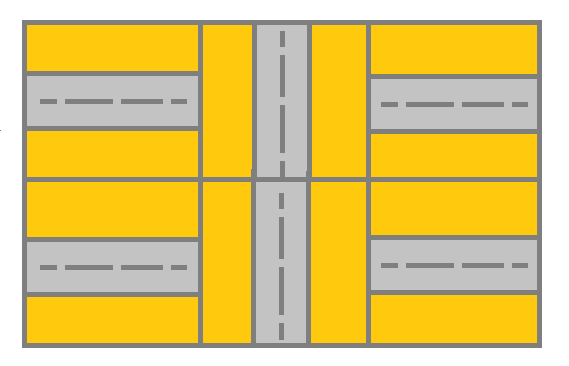1391. Check if There is a Valid Path in a Grid

Given a m x n `grid`. Each cell of the `grid` represents a street. The street of `grid[i][j]` can be:
• 1 which means a street connecting the left cell and the right cell.
• 2 which means a street connecting the upper cell and the lower cell.
• 3 which means a street connecting the left cell and the lower cell.
• 4 which means a street connecting the right cell and the lower cell.
• 5 which means a street connecting the left cell and the upper cell.
• 6 which means a street connecting the right cell and the upper cell.You will initially start at the street of the upper-left cell `(0,0)`. A valid path in the grid is a path which starts from the upper left cell `(0,0)` and ends at the bottom-right cell `(m - 1, n - 1)`. The path should only follow the streets.

Notice that you are not allowed to change any street.

Return true if there is a valid path in the grid or false otherwise.

Example 1:```Input: grid = [[2,4,3],[6,5,2]]
Output: true
Explanation: As shown you can start at cell (0, 0) and visit all the cells of the grid to reach (m - 1, n - 1).
```

Example 2:```Input: grid = [[1,2,1],[1,2,1]]
Output: false
Explanation: As shown you the street at cell (0, 0) is not connected with any street of any other cell and you will get stuck at cell (0, 0)
```

Example 3:

```Input: grid = [[1,1,2]]
Output: false
Explanation: You will get stuck at cell (0, 1) and you cannot reach cell (0, 2).
```

Example 4:

```Input: grid = [[1,1,1,1,1,1,3]]
Output: true
```

Example 5:

```Input: grid = [,,,,,,]
Output: true
```

Constraints:

• `m == grid.length`
• `n == grid[i].length`
• `1 <= m, n <= 300`
• `1 <= grid[i][j] <= 6`

1391. Check if There is a Valid Path in a Grid
``````struct Solution;

use std::collections::VecDeque;

trait Connect {
fn top(self) -> bool;
fn left(self) -> bool;
fn bottom(self) -> bool;
fn right(self) -> bool;
}

impl Connect for i32 {
fn top(self) -> bool {
matches!(self, 2 | 5 | 6)
}
fn left(self) -> bool {
matches!(self, 1 | 3 | 5)
}
fn bottom(self) -> bool {
matches!(self, 2 | 3 | 4)
}
fn right(self) -> bool {
matches!(self, 1 | 4 | 6)
}
}

impl Solution {
fn has_valid_path(grid: Vec<Vec<i32>>) -> bool {
let n = grid.len();
let m = grid.len();
let mut visited = vec![vec![false; m]; n];
let mut queue: VecDeque<(usize, usize)> = VecDeque::new();
queue.push_back((0, 0));
while let Some((i, j)) = queue.pop_front() {
visited[i][j] = true;
if visited[n - 1][m - 1] {
return true;
}
if i > 0 && !visited[i - 1][j] && grid[i][j].top() && grid[i - 1][j].bottom() {
queue.push_back((i - 1, j));
}
if j > 0 && !visited[i][j - 1] && grid[i][j].left() && grid[i][j - 1].right() {
queue.push_back((i, j - 1));
}
if i + 1 < n && !visited[i + 1][j] && grid[i][j].bottom() && grid[i + 1][j].top() {
queue.push_back((i + 1, j));
}
if j + 1 < m && !visited[i][j + 1] && grid[i][j].right() && grid[i][j + 1].left() {
queue.push_back((i, j + 1));
}
}
false
}
}

#[test]
fn test() {
let grid = vec_vec_i32![[2, 4, 3], [6, 5, 2]];
let res = true;
assert_eq!(Solution::has_valid_path(grid), res);
let grid = vec_vec_i32![[1, 2, 1], [1, 2, 1]];
let res = false;
assert_eq!(Solution::has_valid_path(grid), res);
let grid = vec_vec_i32![[1, 1, 2]];
let res = false;
assert_eq!(Solution::has_valid_path(grid), res);
let grid = vec_vec_i32![[1, 1, 1, 1, 1, 1, 3]];
let res = true;
assert_eq!(Solution::has_valid_path(grid), res);
let grid = vec_vec_i32![, , , , , , ];
let res = true;
assert_eq!(Solution::has_valid_path(grid), res);
}
``````